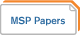Welcome, you are visiting Aspire

Project Aspire: Defining and Assessing Mathematical Knowledge For Teaching Secondary Mathematics
This project focuses on mathematical knowledge for teaching at the secondary level, building upon and extending existing work for elementary mathematics. The project is developing two related assessment instruments: one for assessing teachers' mathematical knowledge for teaching secondary mathematics (MKTsm) and one for assessing the quality of secondary mathematics instruction (IQAsm). Used simultaneously, these instruments are capable of linking secondary teachers' mathematical knowledge with their instruction. The first instrument draws from prior research on teachers' and students' understanding of key mathematical ideas. The second extends the Instructional Quality Assessment (IQA), an existing assessment that focuses on the quality of elementary and middle school mathematics, so that it has a clearer focus on secondary mathematics and on the quality of mathematical ideas that a teacher's instruction fosters.

### Featured ContentProject ASPIRE: Defining and Assessing Mathematical Knowledge (Meanings) for Teaching Secondary Mathematics
Project Aspire will develop two assessment instruments -- one to assess teachers' Mathematical Knowledge for Teaching Secondary Mathematics and one to assess the quality of instruction in secondary mathematics classrooms.…

### Project ContributionsMathematical Meanings of Korean and USA Mathematics Teachers for Mathematical Ideas They Teach
"There are many studies of teachers' mathematical knowledge for teaching mathematics, mostly at pre-high school levels. In my presentation I will give an overview of the MMTsm and comparative results…Researching Mathematical Meanings For Teaching
"Teachers' mathematical knowledge for teaching is a central focus of recent research on teaching mathematics. At the same time, research on mathematical knowledge for teaching has focused largely on teachers'…A Conceptual Approach To Calculus Made Possible By Technology
"Calculus reform and using technology to teach calculus are two longtime endeavors that appear to have failed to make the differences in student understanding predicted by proponents. We argue that…An Assessment of Teachers' Mathematical Meanings for Teaching Secondary Mathematics and its Implications for MSPs
"This session discusses preliminary results from a project to assess high school teachers' mathematical meanings for teaching secondary mathematics. Instead of assessing the extent to which teachers are able to…A Methodology For Investigating Teachers' Mathematical Meanings For Teaching
"We first argue that a focus on teachers' mathematical meanings rather than on their mathematical knowledge holds greater potential for connecting research on what teachers know, what they do, and…Teachers' Meanings For Function Notation
"This study explores teachers' meanings for function notation and function definitions. We focus on what teachers see as representing an output and as representing a function definition, and we analysed…Function Notation As An Idiom
"Functions play a large role in mathematics education beginning in middle school. The aim of this paper is to investigate the meaning teachers hold for function notation; namely, we suggest…Secondary Teachers' Relative Size Schemes
"This paper explores the usefulness of understanding quotients as measures of relative size in mathematics. The paper characterizes the types of thinking displayed by high school mathematics teachers on two…Project ASPIRE: Defining and Assessing Mathematical Knowledge (Meanings) for Teaching Secondary Mathematics
Posted by: Patrick Thompson . Project Aspire will develop two assessment instruments -- one to assess teachers' Mathematical Knowledge for Teaching Secondary Mathematics and one to assess the quality of instruction in secondary mathematics classrooms.…